Recently I found an interesting dataset with indicators for the game broken down by a number of indicators that were recorded before and after the innovation. It was suggested to make a comparison of how the new technology had an impact on the product. To do this, I decided to use the AB test. Based on the analysis, it is necessary to make a business decision on whether to adhere to the baseline (option A) or promote option B.

To get started, let's import our libraries.

```import pandas as pd
import numpy as np
import seaborn as sns

from scipy.stats import mannwhitneyu
from scipy.stats import ttest_ind
from scipy.stats import norm
from scipy.stats import shapiro
from scipy.stats import levene
from scipy.stats import normaltest

import matplotlib.pyplot as plt
```

Create a function for bootstrap

```def get_bootstrap(
data_column_1, # numeric values of the first sample
data_column_2, # numeric values of the second sample
boot_it = 10000, # number of bootstrap subsamples
statistic = np.mean, # statistics of interest to us
bootstrap_conf_level = 0.95 # significance level
):
boot_len = max([len(data_column_1), len(data_column_2)])
boot_data = []
for i in range(boot_it): # extracting subsamples
samples_1 = data_column_1.sample(
boot_len,
replace = True # return parameter
).values

samples_2 = data_column_2.sample(
boot_len, # to preserve the variance, we take the same sample size
replace = True
).values

boot_data.append(statistic(samples_1-samples_2))
pd_boot_data = pd.DataFrame(boot_data)

left_quant = (1 - bootstrap_conf_level)/2
right_quant = 1 - (1 - bootstrap_conf_level) / 2
quants = pd_boot_data.quantile([left_quant, right_quant])

p_1 = norm.cdf(
x = 0,
loc = np.mean(boot_data),
scale = np.std(boot_data)
)
p_2 = norm.cdf(
x = 0,
loc = -np.mean(boot_data),
scale = np.std(boot_data)
)
p_value = min(p_1, p_2) * 2

return {"p_value": p_value}
```

### 1. Now, read in the data.

a. Read in the dataset and take a look at the top few rows here:

```game = pd.read_csv('RhinoGames.csv', skiprows=1)
```
A B Unnamed: 2 A.1 B.1 Unnamed: 5 A.2 B.2 Unnamed: 8 Number of sessions A.3 B.3 Unnamed: 12 A.4 B.4
0 3.0 6.0 NaN 1.0 0.0 NaN 1.0 0.0 NaN 1.0 2050.0 2021.0 NaN 800.340000 1833.380
1 6.0 4.0 NaN 0.0 2.0 NaN 5.0 3.0 NaN 2.0 797.0 730.0 NaN 1470.880526 1286.595
2 6.0 0.0 NaN 0.0 0.0 NaN 0.0 0.0 NaN 3.0 440.0 454.0 NaN 1281.303462 67.270
3 6.0 2.0 NaN 0.0 1.0 NaN 3.0 14.0 NaN 4.0 280.0 271.0 NaN 77.630000 1291.180
4 8.0 4.0 NaN 1.0 0.0 NaN 4.0 3.0 NaN 5.0 207.0 212.0 NaN 616.151000 259.750

b. Use the call below to find information about the dataset.

```game.info()
```
```<class 'pandas.core.frame.DataFrame'>
RangeIndex: 2540 entries, 0 to 2539
Data columns (total 15 columns):
#   Column              Non-Null Count  Dtype
---  ------              --------------  -----
0   A                   642 non-null    float64
1   B                   607 non-null    float64
2   Unnamed: 2          0 non-null      float64
3   A.1                 743 non-null    float64
4   B.1                 839 non-null    float64
5   Unnamed: 5          0 non-null      float64
6   A.2                 2115 non-null   float64
7   B.2                 1969 non-null   float64
8   Unnamed: 8          0 non-null      float64
9   Number of sessions  20 non-null     float64
10  A.3                 20 non-null     float64
11  B.3                 20 non-null     float64
12  Unnamed: 12         0 non-null      float64
13  A.4                 2540 non-null   float64
14  B.4                 2505 non-null   float64
dtypes: float64(15)
memory usage: 297.8 KB
```

c. Separate the various characteristics used for the AB test into separate datasets

```rvaw = game[['A', 'B']][:642]

# Interstitial Ads Watched
iaw = game[['A.1', 'B.1']][:839]
iaw.columns = ['A', 'B']

# User Progress Level
upl = game[['A.2', 'B.2']][:2115]
upl.columns = ['A', 'B']

# Daily Session Number
dsn = game[['A.3', 'B.3']][:20]
dsn.columns = ['A', 'B']

# Session Duration (in seconds)
sd = game[['A.4', 'B.4']][:2540]
sd.columns = ['A', 'B']
```

### 2. Checking the distribution

#### 2.1 Normality Assumption (Shapiro Test)

• H0: Normal distribution assumption is provided.
• H1: ... not provided.
##### 2.1.1. Rewarded Videos Ads Watched
```shapiro(rvaw['A'].dropna())
```
`ShapiroResult(statistic=0.8503138422966003, pvalue=3.579607029873238e-24)`

Based on the Shapiro-Wilkes test, it can be concluded that the distribution of data Rewarded Videos Ads Watched (A) differs significantly from normal, because pvalue = 3.58e-24 < 0.05.

```shapiro(rvaw['B'].dropna())
```
`ShapiroResult(statistic=0.8587424159049988, pvalue=6.443850062887458e-23)`

Based on the Shapiro-Wilkes test, it can be concluded that the distribution of data Rewarded Videos Ads Watched (B) differs significantly from normal, because pvalue = 6.44e-23 < 0.05.

##### 2.1.2. Interstitial Ads Watched
```shapiro(iaw['A'].dropna())
```
`ShapiroResult(statistic=0.7230092287063599, pvalue=2.628179820460071e-33)`

Based on the Shapiro-Wilkes test, it can be concluded that the distribution of data Interstitial Ads Watched (A) differs significantly from normal, because pvalue = 2.63e-33 < 0.05.

```shapiro(iaw['B'].dropna())
```
`ShapiroResult(statistic=0.8238729238510132, pvalue=2.3491646353386526e-29)`

Based on the Shapiro-Wilkes test, it can be concluded that the distribution of data Interstitial Ads Watched (B) differs significantly from normal, because pvalue = 2.35e-29 < 0.05.

##### 2.1.3. Daily Session Number
```shapiro(dsn['A'].dropna())
```
`ShapiroResult(statistic=0.5037522315979004, pvalue=3.4377018209852395e-07)`

Comment: H0 hyptothesis was rejected because pvalue = 3.4377018209852395e-07 < 0.05. Statistically, it could be rejected that the normal distribution assumption of the data set in which the Daily Session Number(A) was measured was met.

```shapiro(dsn['B'].dropna())
```
`ShapiroResult(statistic=0.4918368458747864, pvalue=2.6840217515200493e-07)`

Comment: H0 hyptothesis was rejected because pvalue = 2.6840217515200493e-07 < 0.05. Statistically, it could be rejected that the normal distribution assumption of the data set in which the Daily Session Number(B) was measured was met.

#### 2.2. Variance Homogeneity Assumption (Levene Testi)

• H0 : Variances are homogeneous.
• H1 : Variances are not homogeneous.
##### 2.2.1. Rewarded Videos Ads Watched
```levene(rvaw['A'].dropna(), rvaw['B'].dropna())
```
`LeveneResult(statistic=0.011173214244696214, pvalue=0.915834662474728)`

Comment: Since the pvalue = 0.92 > 0.05, the H0 hypothesis, that is, the variances were not statistically rejected as homogeneous.

##### 2.2.2. Interstitial Ads Watched
```levene(iaw['A'].dropna(), iaw['B'].dropna())
```
`LeveneResult(statistic=161.25546237868772, pvalue=2.9805577740785094e-35)`

Comment: Since the pvalue = 2.98e-35 < 0.05, the H0 hypothesis, that is, the variances were statistically rejected as homogeneous.

##### 2.2.3. User Progress Level
```levene(upl['A'].dropna(), upl['B'].dropna())
```
`LeveneResult(statistic=55.565871472172205, pvalue=1.0974048105821832e-13)`

Comment: Since the pvalue = 1.10e-13 < 0.05, the H0 hypothesis, that is, the variances were statistically rejected as homogeneous.

##### 2.2.4. Daily Session Number
```levene(dsn['A'].dropna(), dsn['B'].dropna())
```
`LeveneResult(statistic=0.004414099203848678, pvalue=0.9473768992864252)`

Comment: Since the pvalue = 0.95 > 0.05, the H0 hypothesis, that is, the variances were not statistically rejected as homogeneous.

##### 2.2.5. Session Duration (in seconds)
```levene(sd['A'].dropna(), sd['B'].dropna())
```
`LeveneResult(statistic=0.9081732330528205, pvalue=0.34064525235319454)`

Comment: Since the pvalue = 0.95 > 0.05, the H0 hypothesis, that is, the variances were not statistically rejected as homogeneous.

#### 2.3. Descriptive statistics

##### 2.3.1. Rewarded Videos Ads Watched
```rvaw.describe()
```
A B
count 642.000000 607.000000
mean 2.292835 2.319605
std 2.402205 2.383246
min 0.000000 0.000000
25% 0.000000 0.000000
50% 1.000000 2.000000
75% 4.000000 4.000000
max 8.000000 8.000000
```sns.boxplot(data=rvaw)
sns.displot(rvaw, stat="probability")
```
`<seaborn.axisgrid.FacetGrid at 0x7fb66002c670>`##### 2.3.2. Interstitial Ads Watched
```iaw.describe()
```
A B
count 743.000000 839.000000
mean 0.593540 1.346841
std 0.748087 1.497838
min 0.000000 0.000000
25% 0.000000 0.000000
50% 0.000000 1.000000
75% 1.000000 2.000000
max 2.000000 5.000000
```sns.boxplot(data=iaw)
sns.displot(iaw, stat="probability")
```
`<seaborn.axisgrid.FacetGrid at 0x7fb691e71520>`##### 2.3.3. User Progress Level
```upl.describe()
```
A B
count 2115.000000 1969.000000
mean 1.074704 2.046724
std 2.795979 5.250324
min 0.000000 0.000000
25% 0.000000 0.000000
50% 0.000000 0.000000
75% 1.000000 1.000000
max 31.000000 50.000000
```sns.boxplot(data=upl)
sns.displot(upl, stat="probability")
```
`<seaborn.axisgrid.FacetGrid at 0x7fb692247190>`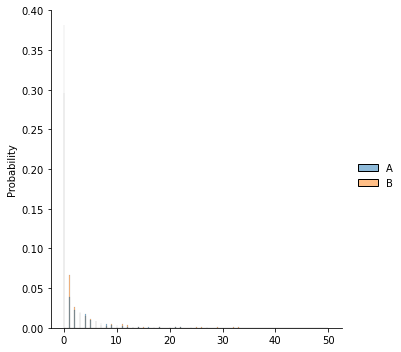##### 2.3.4. Daily Session Number
```dsn.describe()
```
A B
count 20.00000 20.000000
mean 233.00000 226.450000
std 467.51448 457.913974
min 12.00000 12.000000
25% 30.50000 31.250000
50% 65.50000 61.000000
75% 186.00000 161.750000
max 2050.00000 2021.000000
```sns.boxplot(data=dsn)
sns.displot(dsn, stat="probability")
```
`<seaborn.axisgrid.FacetGrid at 0x7fb6b03a0f70>`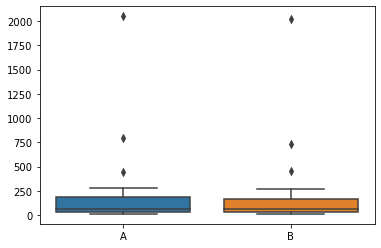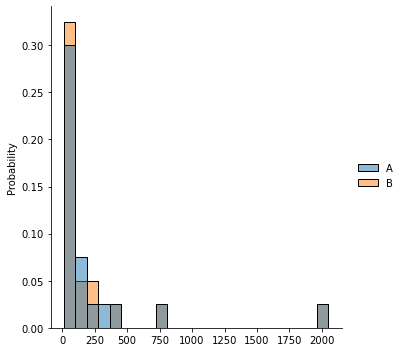##### 2.3.5. Session Duration (in seconds)
```sd.describe()
```
A B
count 2540.000000 2505.000000
mean 708.328796 701.461475
std 746.429697 776.749590
min 10.010000 10.150000
25% 200.150000 176.180000
50% 499.845000 471.310000
75% 960.599038 935.610000
max 10581.530000 7515.430000
```sns.boxplot(data=sd)
sns.displot(sd, stat="probability")
```
`<seaborn.axisgrid.FacetGrid at 0x7fb6929cf100>`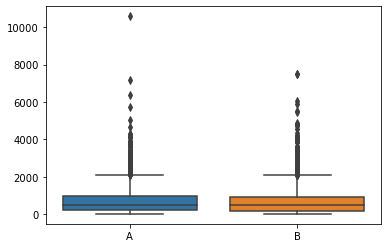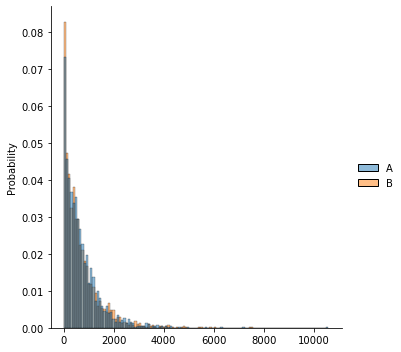### 3. AB Test

• H0 : There is no statistically significant difference between the two groups.
• H1 : ... there is a difference

#### 3.1. T-test:

##### 3.1.1. Rewarded Videos Ads Watched
```ttest_ind(rvaw['A'].dropna(), rvaw['B'].dropna())
```
`Ttest_indResult(statistic=-0.19759680608066377, pvalue=0.8433927375170441)`

Comment: The H0 hypothesis could not be rejected because the result of the T Test was pvalue = 0.84 > 0.05.

So, we could not reject that there was no statistically significant difference between the purchase amounts of the Rewarded Videos Ads Watched (A) and Rewarded Videos Ads Watched (B).

##### 3.1.2. Interstitial Ads Watched
```ttest_ind(iaw['A'].dropna(), iaw['B'].dropna())
```
`Ttest_indResult(statistic=-12.406470258676633, pvalue=8.544370502591074e-34)`

Comment: The H1 hypothesis could not be rejected because the result of the T Test was pvalue = 8.544370502591074e-34 < 0.05.

So, we found a statistically significant difference between the Intermediate Ads Watched (A) and the Intermediate Ads Watched (B).

##### 3.1.3. User Progress Level
```ttest_ind(upl['A'].dropna(), upl['B'].dropna())
```
`Ttest_indResult(statistic=-7.454251905602078, pvalue=1.0974048105809188e-13)`

Comment: The H1 hypothesis could not be rejected because the result of the T Test was pvalue = 1.0974048105809188e-13 < 0.05.

So, we found a statistically significant difference between the User Progress Level (A) and the User Progress Level (B).

##### 3.1.4. Daily Session Number
```ttest_ind(dsn['A'].dropna(), dsn['B'].dropna())
```
`Ttest_indResult(statistic=0.04476154600416851, pvalue=0.964531775418733)`

Comment: The H0 hypothesis could not be rejected because the result of the T Test was pvalue = 0.96 > 0.05.

So, we could not reject that there was no statistically significant difference between the purchase amounts of the Daily Session Number (A) and Daily Session Number (B).

##### 3.1.5. Session Duration (in seconds)
```ttest_ind(sd['A'].dropna(), sd['B'].dropna())
```
`Ttest_indResult(statistic=0.32020668068491875, pvalue=0.7488249250526802)`

Comment: The H0 hypothesis could not be rejected because the result of the T Test was pvalue = 0.78 > 0.05.

So, we could not reject that there was no statistically significant difference between the purchase amounts of the Sassion Duration (A) and Sassion Duration (B).

#### 3.2. Mann-Whitney Rank Criterion

##### 3.2.1. Rewarded Videos Ads Watched
```mannwhitneyu(rvaw['A'].dropna(), rvaw['B'].dropna())
```
`MannwhitneyuResult(statistic=193165.0, pvalue=0.7873798765442723)`

Comment: The H0 hypothesis could not be rejected because the result of the Mann-Whitney Rank Criterion was pvalue = 0.79 > 0.05.

So, we could not reject that there was no statistically significant difference between the purchase amounts of the Rewarded Videos Ads Watched (A) and Rewarded Videos Ads Watched (B).

##### 3.2.2. Interstitial Ads Watched
```mannwhitneyu(iaw['A'].dropna(), iaw['B'].dropna())
```
`MannwhitneyuResult(statistic=231089.0, pvalue=1.5014095689910844e-21)`

Comment: The H1 hypothesis could not be rejected because the result of the Mann-Whitney Rank Criterion was pvalue = 1.5014095689910844e-21 < 0.05.

So, we found a statistically significant difference between the Intermediate Ads Watched (A) and the Intermediate Ads Watched (B).

##### 3.2.3. User Progress Level
```mannwhitneyu(upl['A'].dropna(), upl['B'].dropna())
```
`MannwhitneyuResult(statistic=1830608.5, pvalue=7.967988711774177e-16)`

Comment: The H1 hypothesis could not be rejected because the result of the Mann-Whitney Rank Criterion was pvalue = 7.967988711774177e-16 < 0.05.

So, we found a statistically significant difference between the User Progress Level (A) and the User Progress Level (B).

##### 3.2.4. Daily Session Number
```mannwhitneyu(dsn['A'].dropna(), dsn['B'].dropna())
```
`MannwhitneyuResult(statistic=195.5, pvalue=0.9138246828586165)`

Comment: The H0 hypothesis could not be rejected because the result of the Mann-Whitney Rank Criterion was pvalue = 0.96 > 0.05.

So, we could not reject that there was no statistically significant difference between the purchase amounts of the Daily Session Number (A) and Daily Session Number (B).

##### 3.2.5. Session Duration (in seconds)
```mannwhitneyu(sd['A'].dropna(), sd['B'].dropna())
```
`MannwhitneyuResult(statistic=3274043.5, pvalue=0.07312952222964135)`

Comment: The H0 hypothesis could not be rejected because the result of the Mann-Whitney Rank Criterion was pvalue = 0.07 > 0.05.

So, we could not reject that there was no statistically significant difference between the purchase amounts of the Session Duration (A) and Session Duration (B).

#### 3.3. Bootstrap

##### 3.3.1. Rewarded Videos Ads Watched
```get_bootstrap(rvaw['A'].dropna(), rvaw['B'].dropna())
```
`{'p_value': 0.8382250742144183}`

Comment: The H0 hypothesis could not be rejected because the result of the Bootstrap was pvalue = 0.83 > 0.05.

So, we could not reject that there was no statistically significant difference between the purchase amounts of the Rewarded Videos Ads Watched (A) and Rewarded Videos Ads Watched (B).

##### 3.3.2. Interstitial Ads Watched
```get_bootstrap(iaw['A'].dropna(), iaw['B'].dropna())
```
`{'p_value': 6.769229848823528e-39}`

Comment: The H1 hypothesis could not be rejected because the result of the Bootstrap was pvalue = 4.805207678906211e-39 < 0.05.

So, we found a statistically significant difference between the Intermediate Ads Watched (A) and the Intermediate Ads Watched (B).

##### 3.3.3. User Progress Level
```get_bootstrap(upl['A'].dropna(), upl['B'].dropna())
```
`{'p_value': 4.645521368030348e-14}`

Comment: The H1 hypothesis could not be rejected because the result of the Bootstrap was pvalue = 2.69955949765377e-14 < 0.05.

So, we found a statistically significant difference between the User Progress Level (A) and the User Progress Level (B).

##### 3.3.4. Daily Session Number
```get_bootstrap(dsn['A'].dropna(), dsn['B'].dropna())
```
`{'p_value': 0.956434921092212}`

Comment: The H0 hypothesis could not be rejected because the result of the Bootstrap was pvalue = 0.96 > 0.05.

So, we could not reject that there was no statistically significant difference between the purchase amounts of the Daily Session Number (A) and Daily Session Number (B).

##### 3.3.5. Session Duration (in seconds)
```get_bootstrap(sd['A'].dropna(), sd['B'].dropna())
```
`{'p_value': 0.7422441919911926}`

Comment: The H0 hypothesis could not be rejected because the result of the Bootstrap was pvalue = 0.75 > 0.05.

So, we could not reject that there was no statistically significant difference between the purchase amounts of the Session Duration (A) and Session Duration (B).

### 4. Recommendation to Client

As a result of the analysis of the presented data, it was revealed that it is statistically impossible to confirm that their distribution is normal. In this connection, the comparison of samples was carried out using nonparametric methods. Statistically significant difference was found in Interstitial Ads Watched and User Progress Level. At the same time, the increase in the number of Interstitial Ads Watched did not affect the Daily Session Number and Session Duration (in seconds), which indicates the absence of a negative effect. The statistically significant increase in User Progress Level is probably due to the fact that the sample of users did not change. Also, in both cases, there is an increase in the spread of data.